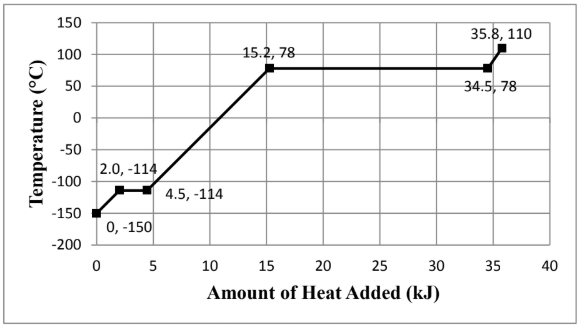# Problem: Consider the heating curve shown below for 22.86 grams of an unknown substance. You may assume constant pressure conditions. Note: the points are labeled as (amount of heat added, temperature). Given that the heat capacity at constant pressure for the liquid is 112.4 J/mol∙K, calculate how many moles of the compound are present in the sample being heated. Show all work and circle your final answer.

###### FREE Expert Solution

We are asked to calculate how many moles of the compound are present in the sample being heated given the heat capacity for the liquid and the heating diagram.

We go through the following steps to solve this problem:

Step 1. Calculate the amount of heat added based on the heating curve

Step 2. Calculate the mole of the substance###### Problem Details

Consider the heating curve shown below for 22.86 grams of an unknown substance. You may assume constant pressure conditions. Note: the points are labeled as (amount of heat added, temperature).Given that the heat capacity at constant pressure for the liquid is 112.4 J/mol∙K, calculate how many moles of the compound are present in the sample being heated. Show all work and circle your final answer.